# 12. Convert to polar coordinates and evaluate: 1 LL62 +7) dydx + y2 +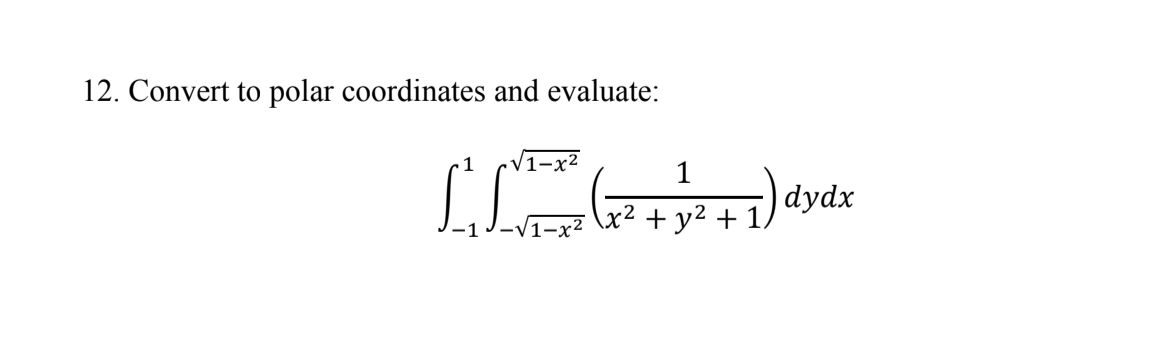12. Convert to polar coordinates and evaluate: 1 LL62 +7) dydx + y2 +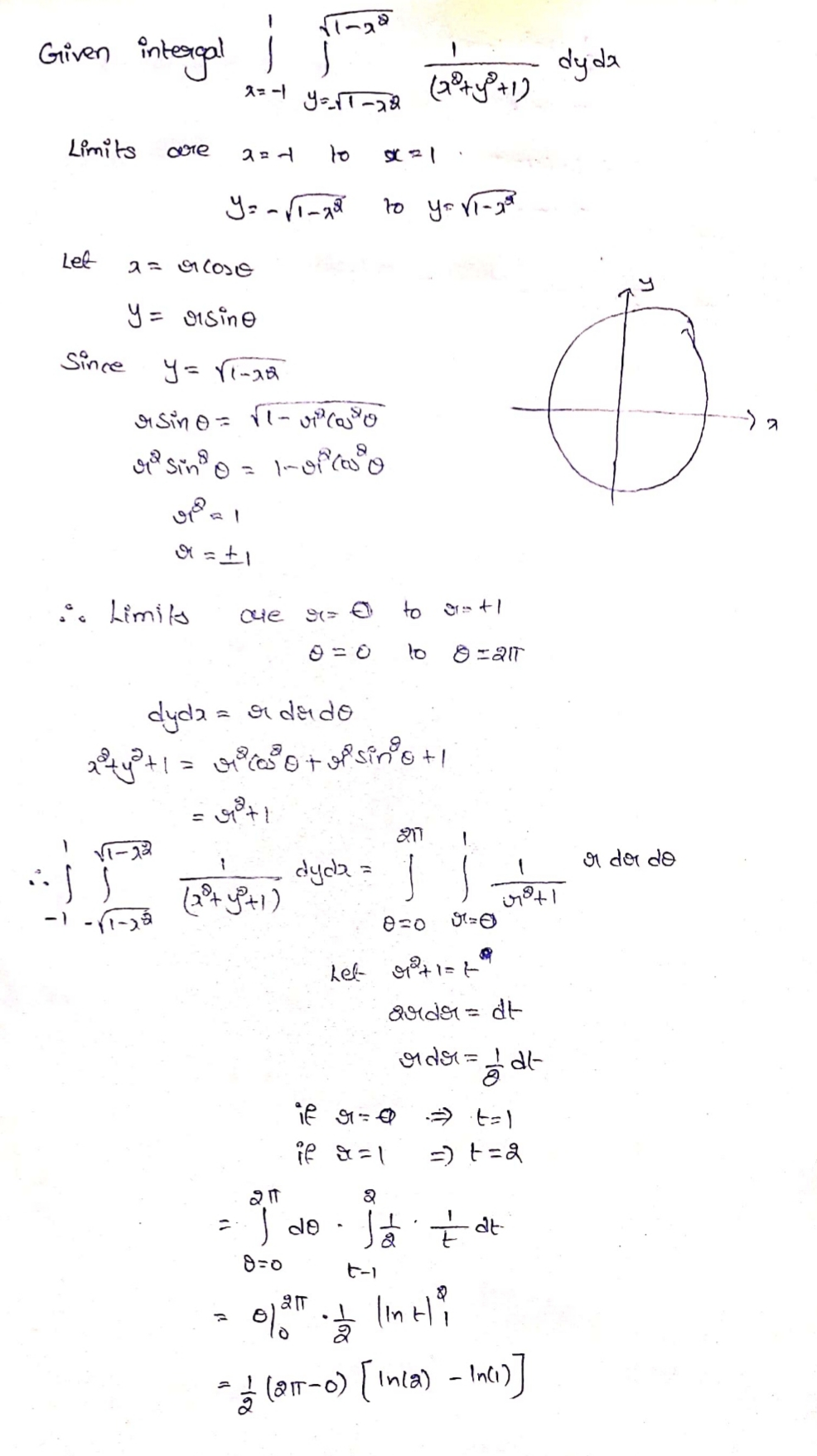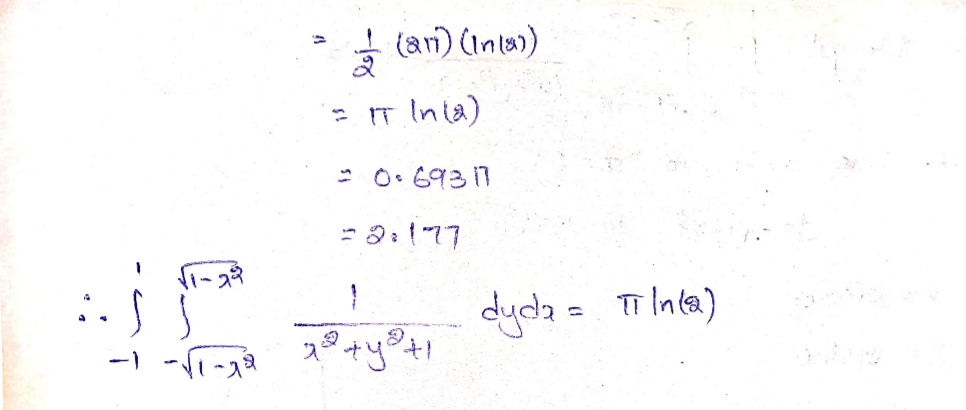##### Add Answer of: 12. Convert to polar coordinates and evaluate: 1 LL62 +7) dydx + y2 +
Similar Homework Help Questions
• ### Using polar coordinates, evaluate the integral so Som x(x2 + y²) dydx. Be careful to check...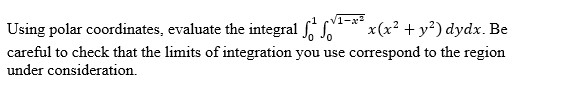Using polar coordinates, evaluate the integral so Som x(x2 + y²) dydx. Be careful to check that the limits of integration you use correspond to the region under consideration.

• ### polar coordinates

given the iterated integral of ( xy)dydx from from 0 to (x+7) , then integral of the result from 0 to 3 . convert it to polar coordinates.

• ### Convert the equation to polar coordinates. x2 + y2 = 7y + X Sketch the graph.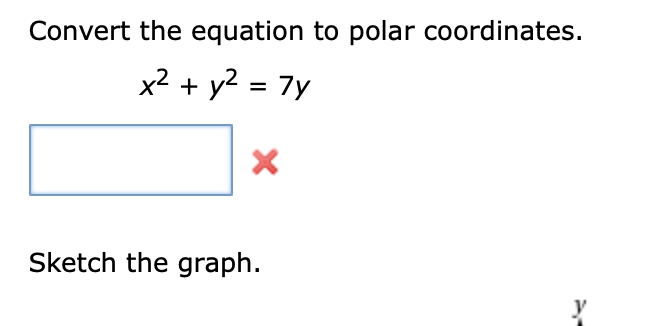Convert the equation to polar coordinates. x2 + y2 = 7y + X Sketch the graph.

• ### onvert to pola coordinates

given the integral of (xy)dydx from 0 to (x+7) then integral of the resulting integral from 0 to 3 .(a) convert it to polar coordinates.(b) evaluate the polar - coordinates- integral in part (a).

• ### 11. Use polar coordinates to evaluate the integral 1,8-2V(+ y2)<dy dx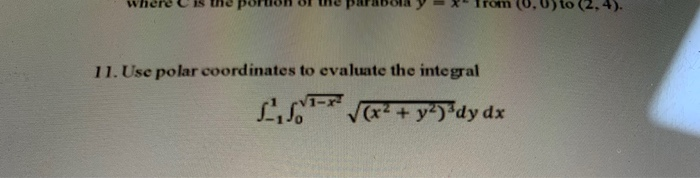11. Use polar coordinates to evaluate the integral 1,8-2V(+ y2)<dy dx

• ### Problem #2: Evaluate the following by changing to polar coordinates. 81 - y2 81 - y2...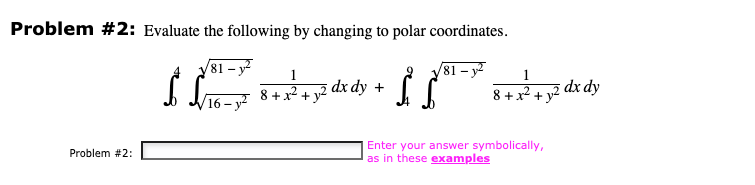Problem #2: Evaluate the following by changing to polar coordinates. 81 - y2 81 - y2 8 + x2 + y2 dx dy + 8 + x2 + 16 - y2 Problem #2: Enter your answer symbolically, as in these examples

• ### Double Intergals in Polar Coordinates: 4. Use polar coordinates to find the volume of the solid...

Double Intergals in Polar Coordinates: 4. Use polar coordinates to find the volume of the solid that is bounded by the paraboloids z = 3x^2 + 3y^2 and z = 4 ? x^2 ? y^2. 5. Evaluate by converting to polar coordinates ? -3 to3 * ? 0 to sqrt(9-x^2) (sin(x^2 +y^2) dydx 6. Evaluate by converting to polar coordinates: ? 0 to 1 * ? -sqrt(1-y^2) to 0 (x^2(y)) dxdy

• ### 9. [-/1 Points] DETAILS SALGTRIG4 8.1.041. Convert the rectangular coordinates to polar coordinates with r>0 and...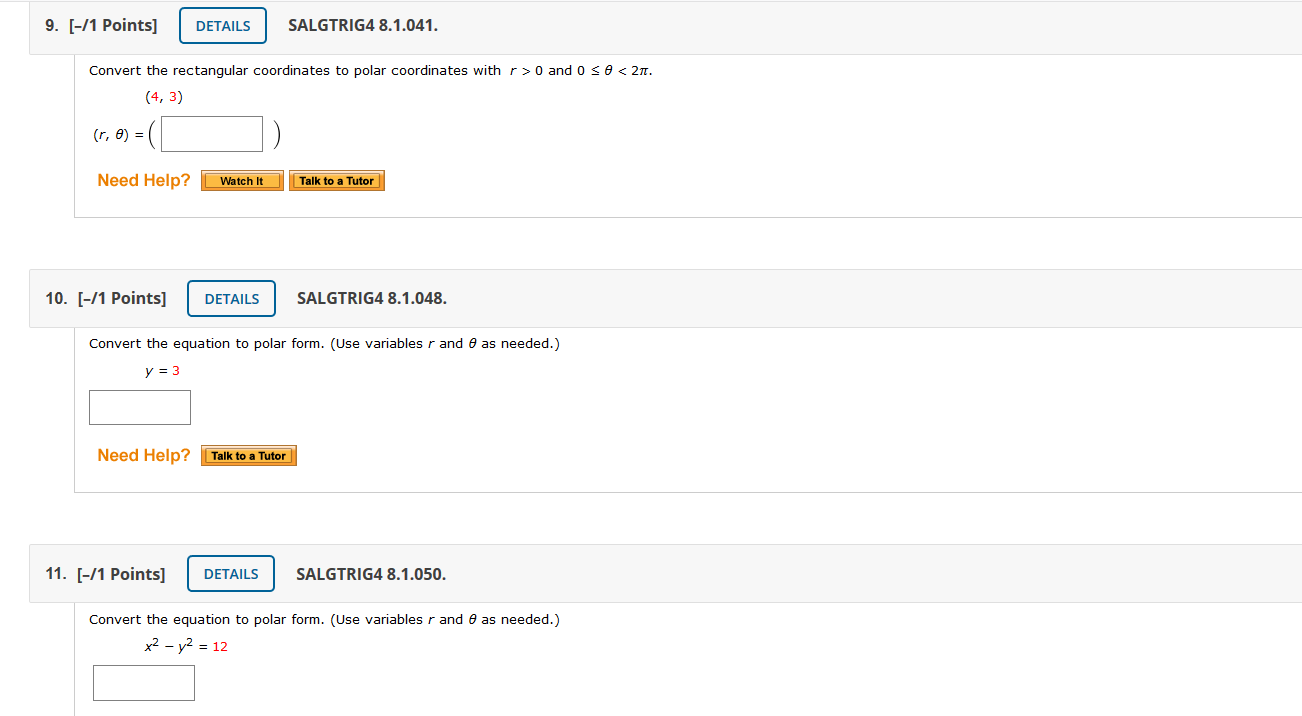9. [-/1 Points] DETAILS SALGTRIG4 8.1.041. Convert the rectangular coordinates to polar coordinates with r>0 and 0 se<27. (4,3) (r, 0) = =(. Need Help? Watch It Talk to a Tutor 10. [-/1 Points] DETAILS SALGTRIG4 8.1.048. Convert the equation to polar form. (Use variables r and as needed.) y = 3 Need Help? Talk to a Tutor 11. [-/1 Points] DETAILS SALGTRIG4 8.1.050. Convert the equation to polar form. (Use variables r and e as needed.) x2 - y2...

• ### U 12. [-/1 Points] DETAILS SALGTRIG4 8.1.057. Convert the polar equation to rectangular coordinates. (Use variables...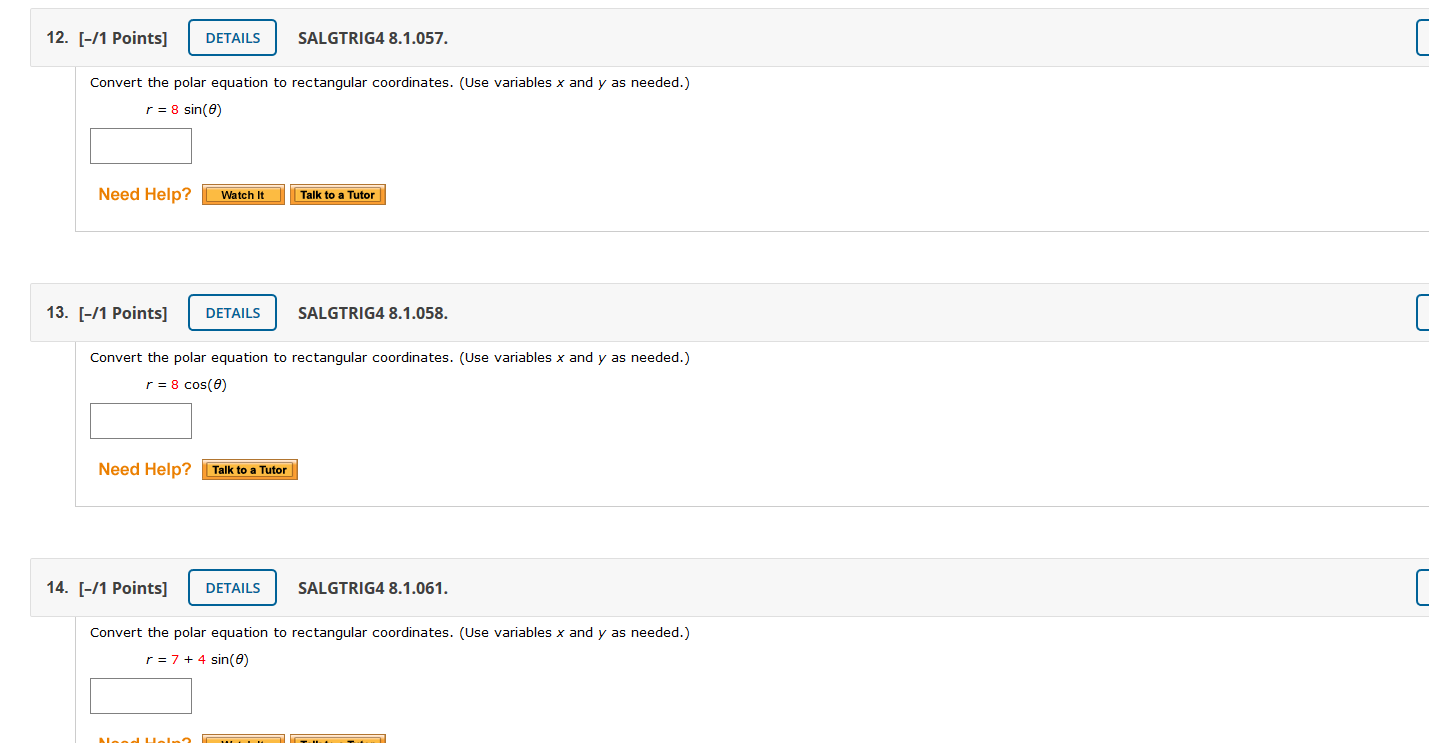U 12. [-/1 Points] DETAILS SALGTRIG4 8.1.057. Convert the polar equation to rectangular coordinates. (Use variables x and y as needed.) r = 8 sin(e) Need Help? Watch It Talk to a Tutor 13. [-/1 Points] DETAILS SALGTRIG4 8.1.058. Convert the polar equation to rectangular coordinates. (Use variables x and y as needed.) r = 8 cos(0) Need Help? Talk to a Tutor U 14. [-/1 Points] DETAILS SALGTRIG4 8.1.061. Convert the polar equation to rectangular coordinates. (Use variables x...

• ### Plot the point given by the polar coordinates. 1 (19) 2. Convert each point from polar...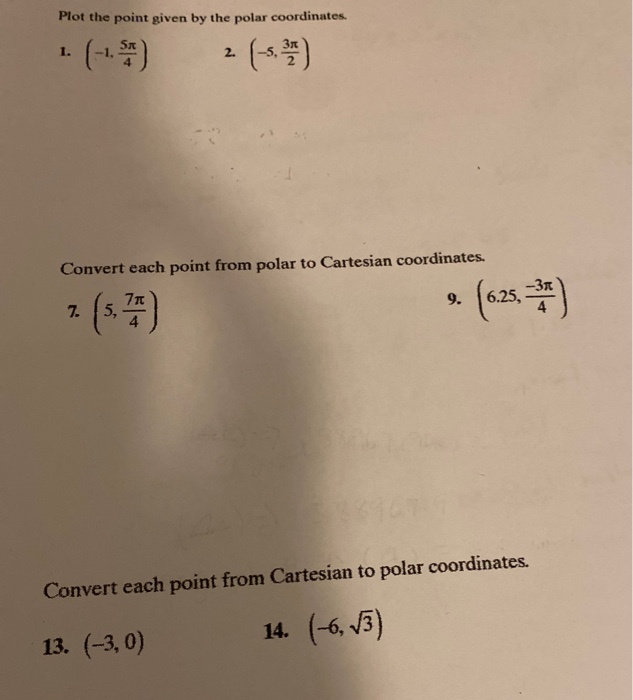Plot the point given by the polar coordinates. 1 (19) 2. Convert each point from polar to Cartesian coordinates. -Зл 71 7. 5, 9. 6.25, 3,7) Convert each point from Cartesian to polar coordinates. 14. (-6, V3) 13. (-3,0)

Free Homework App